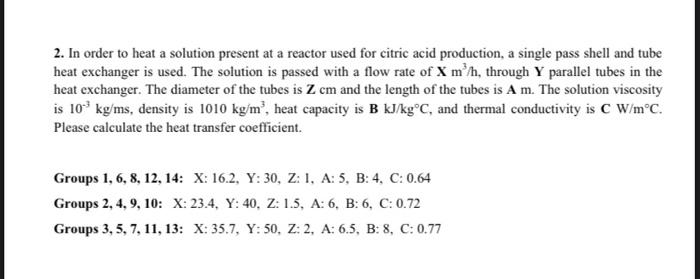Home / Expert Answers / Chemical Engineering / 2-in-order-to-heat-a-solution-present-at-a-reactor-used-for-citric-acid-production-a-single-pass-pa306

# (Solved): 2. In order to heat a solution present at a reactor used for citric acid production, a single pass ...2. In order to heat a solution present at a reactor used for citric acid production, a single pass shell and tube heat exchanger is used. The solution is passed with a flow rate of $$\mathbf{X} \mathrm{m}^{3} / \mathrm{h}$$, through $$\mathbf{Y}$$ parallel tubes in the heat exchanger. The diameter of the tubes is $$\mathbf{Z} \mathbf{~ c m}$$ and the length of the tubes is $$\mathbf{A} \mathbf{m}$$. The solution viscosity is $$10^{-3} \mathrm{~kg} / \mathrm{ms}$$, density is $$1010 \mathrm{~kg} / \mathrm{m}^{3}$$, heat capacity is $$\mathbf{B ~ k J} / \mathrm{kg}^{\circ} \mathrm{C}$$, and thermal conductivity is $$\mathbf{C} \mathrm{W} / \mathrm{m}^{\circ} \mathrm{C}$$. Please calculate the heat transfer coefficient.

We have an Answer from Expert